## Thursday, May 30, 2019

 table div table+table+table div table{width:100%;padding:0}table div table+table+table div table img{width:96.23%;padding:0;float:none}table div table+table+table div table td{width:100%;padding:0 1.88% 18px}/* styles */# Sunday's Service - June 2, 2019

 /* styles */ We welcome back Phil Bartholomew to lead us in Worship this week. The featured Scripture verses are: Acts 1:1-26 for Phil's message: Seeing Spots.
 /* styles */ ~~~

# CG NEWS & EVENTS## Bible Studies

Sunday Morning Class: Romans, 9:30a.m.

Thursday Morning Ladies Study: Hosea, 9:00am at the church (We have had a change of location, day, and time for the summer. Next meeting will be June 13.)

You are welcome to join in studies at any time!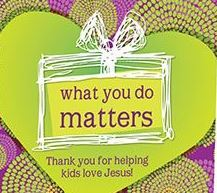## Garden & Nursery News

Thank you to all our leaders who helped us have another terrific year of Children's Ministry! The Garden is on Summer Break until September 8th.Activity pages will be on the table by the Sanctuary doors. Children 3 & younger are invited to the Nursery for Bible story time.

## Youth Group

Summer plans will be on the schedule by next week.

 table div table+table+table+table+table+table+table+table+table+table+table+table div table{width:100%;padding:0}table div table+table+table+table+table+table+table+table+table+table+table+table div table img{width:96.23%;padding:0;float:none}table div table+table+table+table+table+table+table+table+table+table+table+table div table td{width:100%;padding:0 1.88% 18px}/* styles */# UPCOMING AT CG## June Speakers

June 9 - Phil Bartholomew
June 16 - John Muckridge
June 30 - Adam Shank - Communion Sunday

## Welcome Summer Potluck

June 23 After Worship
Hot dogs with all the fixin's and an ice cream sundae bar provided. Dust off your favorite summer recipes and bring a dish to share. Sign up sheet on the bulletin board.

 table div table+table+table+table+table+table+table+table+table+table+table+table+table+table+table+table div table{width:100%;padding:0}table div table+table+table+table+table+table+table+table+table+table+table+table+table+table+table+table div table img{width:96.23%;padding:0;float:none}table div table+table+table+table+table+table+table+table+table+table+table+table+table+table+table+table div table td{width:100%;padding:0 1.88% 18px}/* styles *//* styles */ www.goodnessgrows4all.org Our Mission Statement: To cultivate personal, social, and community growth through gardening, education, and opportunity for people of all abilities. ~~~

# NEWS & EVENTS

## H&H Safety Day 2019

Rachel & Sue will have a butterfly craft for kids and samples of some of our projects at this fantastic community day celebrating everyday heroes.

## 8th Annual RiverFest

Kitty & Jen will be hanging out by the river with Jungle Jam, Juicy Jam, and ferns to purchase. So much to see, right in downtown Youngstown!## Ferns Still Available

Boston ferns, limited supply, \$18 + tax. Buy some beauty for your home, porch, or yard while raising money to support the work of Goodness Grows. Check them out in the greenhouse on Sunday or stop by during the week. Please spread the word to your friends and family about our ferns and the work we do here at GG!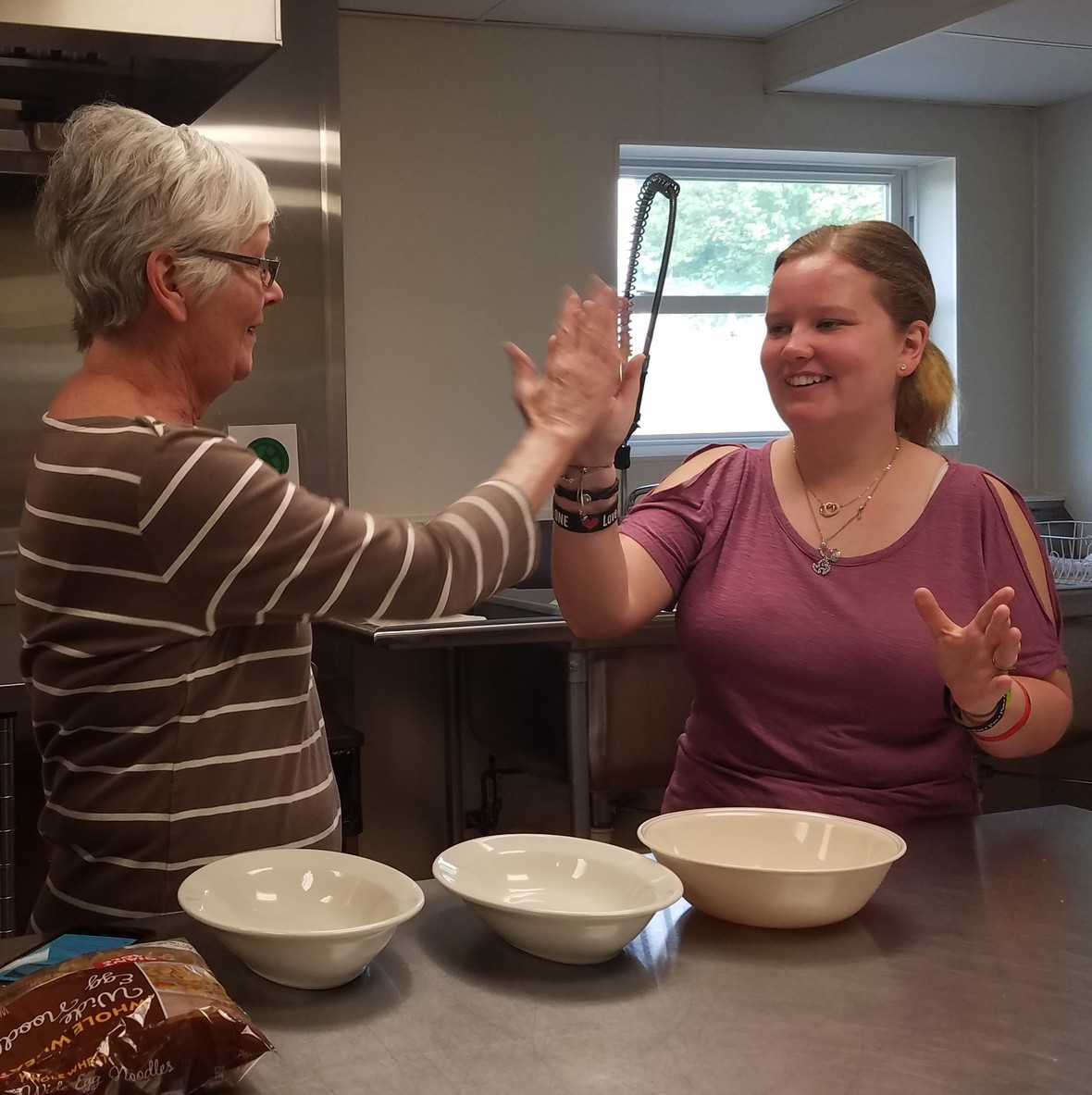Celebrating Good Work

## Summer at GG

Since GG was closed on Monday, we kicked off our week with Sue Weaver and our Tuesday cooking project. Dustin, Tori, and Sue made beef stroganoff and homemade cinnamon applesauce. Yum! Everyone loves Tuesdays here!
The rest of the week wasn't as tasty, but it was definitely busy. We made jam, worked in the greenhouse and warehouse, and, of course, dodged raindrops to get some important outside tasks done. We are quite ready for the rain to go away so we can spend more time in the gardens and raised beds! Our CSA pick up days will be here before we know it!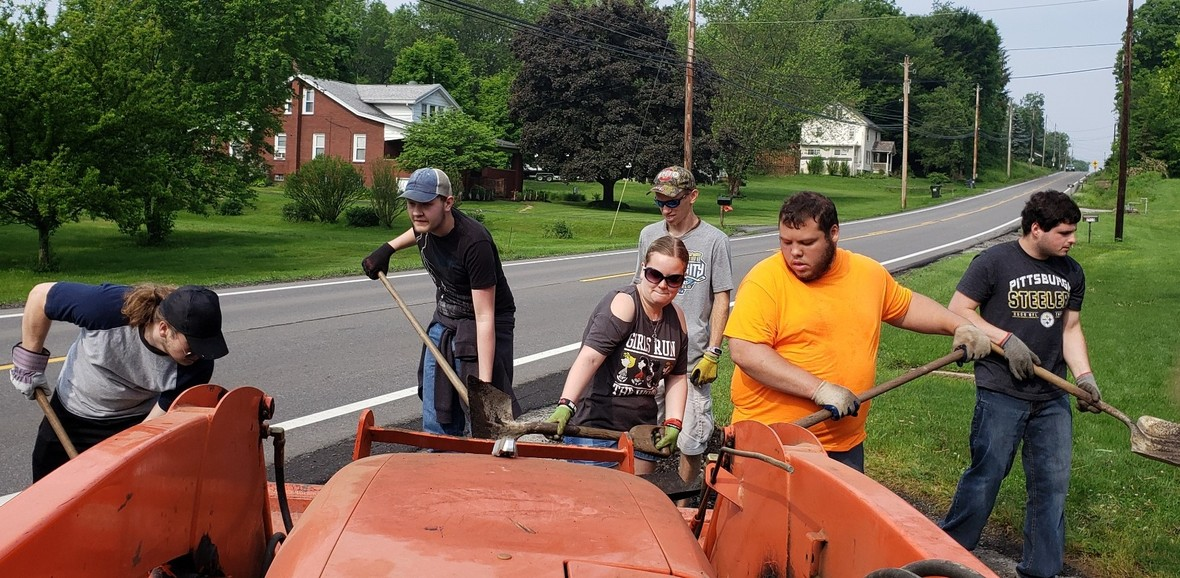Storm Cleanup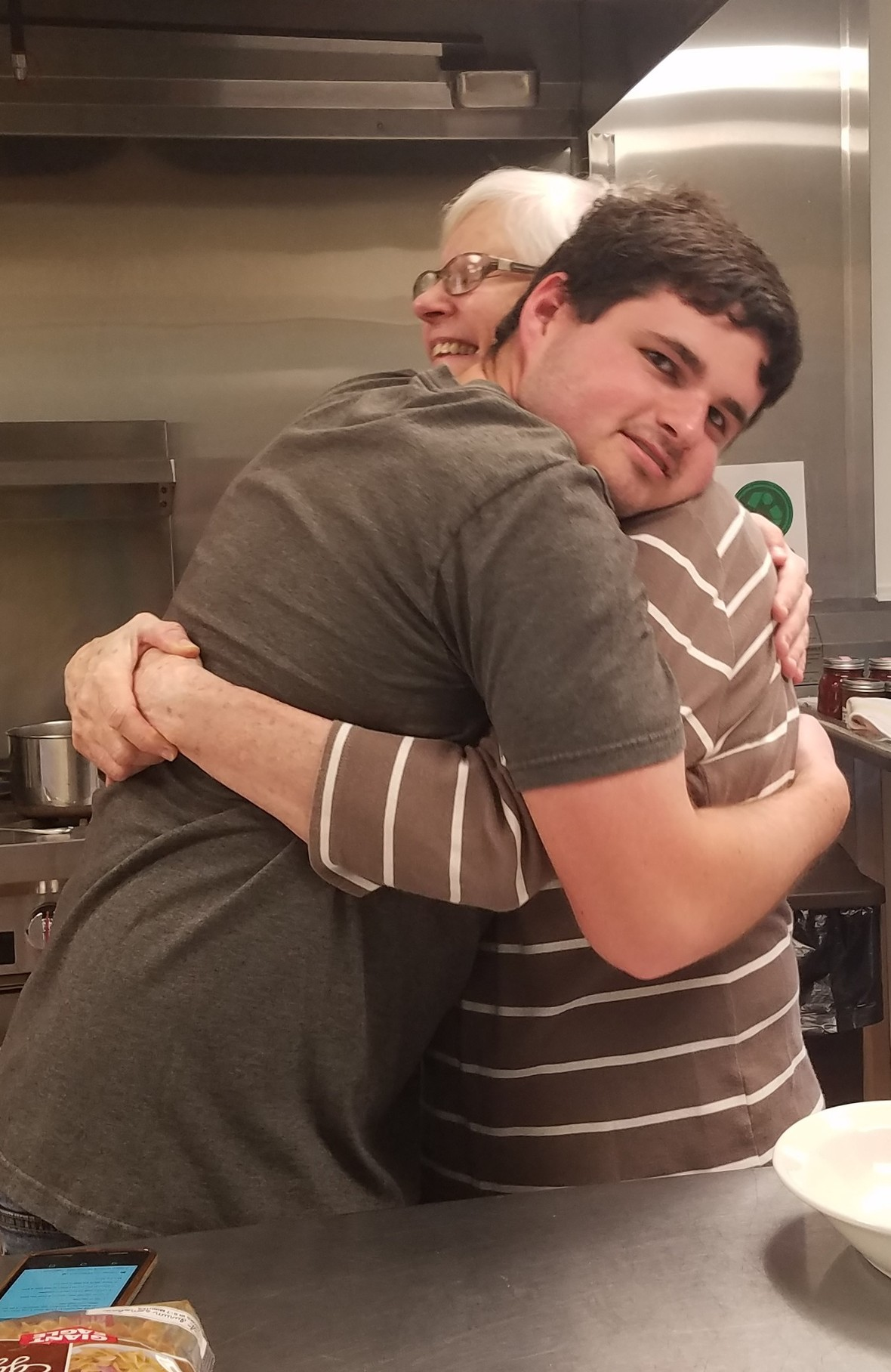Cooking with Mrs. Weaver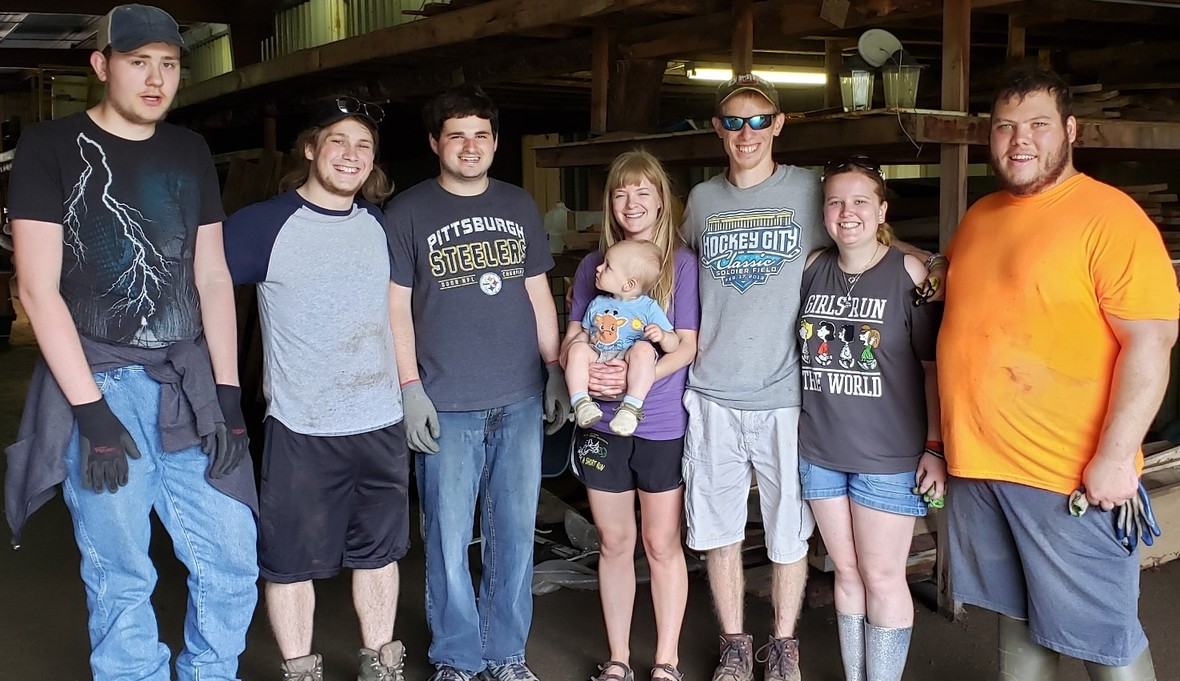Claire Visited!
 table div table+table+table+table+table+table+table+table+table+table+table+table+table+table+table+table+table+table+table+table+table+table+table+table+table+table div table{width:100%;padding:0}table div table+table+table+table+table+table+table+table+table+table+table+table+table+table+table+table+table+table+table+table+table+table+table+table+table+table div table img{width:96.23%;padding:0;float:none}table div table+table+table+table+table+table+table+table+table+table+table+table+table+table+table+table+table+table+table+table+table+table+table+table+table+table div table td{width:100%;padding:0 1.88% 18px}/* styles */# OUTREACH and MISSION OPPORTUNITIES## Coat & Clothing Drive

Ongoing Outreach/Mission Opportunity
Drop items off in the collection barrel or take them up to the Green Room, anytime throughout the year! Let your friends and family know that we accept donations year-round. We are happy to take coats, clothes, shoes, purses and bags, jewelry, hats, gloves and mittens, scarves, and even small household items. Anything that is clean and in good repair that you think someone else might be able to use!

Date for Giveaway Set ~ Saturday, October 12th, 10am-1pm!

 /* styles */ ~~
 /* styles */ For I was hungry and you gave me food; I was thirsty and you gave me a drink; I was a stranger and you took me in; I was naked and you clothed me; I was sick and you visited me. I was in prison and you came to me. .....When you did it to one of the least of these my brothers and sisters, you were doing it to me. Matthew 25: 35-36, 40 ~ What can YOU do?
 table div table+table+table+table+table+table+table+table+table+table+table+table+table+table+table+table+table+table+table+table+table+table+table+table+table+table+table+table+table+table+table div table{width:100%;padding:0}table div table+table+table+table+table+table+table+table+table+table+table+table+table+table+table+table+table+table+table+table+table+table+table+table+table+table+table+table+table+table+table div table img{width:96.23%;padding:0;float:none}table div table+table+table+table+table+table+table+table+table+table+table+table+table+table+table+table+table+table+table+table+table+table+table+table+table+table+table+table+table+table+table div table td{width:100%;padding:0 1.88% 18px}/* styles */# LOCAL COMMUNITY NEWS/EVENTS

 /* styles */ If you know of any interesting news or events, please let us know, so that we can pass it on to others!

## North Lima News

 table.module-34{width:0%;padding:0}table div table+table+table+table+table+table+table+table+table+table+table+table+table+table+table+table+table+table+table+table+table+table+table+table+table+table+table+table+table+table+table+table+table+table+table div table{width:0%;float:none;margin-left:auto;margin-right:auto;padding:0}table div table+table+table+table+table+table+table+table+table+table+table+table+table+table+table+table+table+table+table+table+table+table+table+table+table+table+table+table+table+table+table+table+table+table+table div table a{border:0 none;text-decoration:none}table div table+table+table+table+table+table+table+table+table+table+table+table+table+table+table+table+table+table+table+table+table+table+table+table+table+table+table+table+table+table+table+table+table+table+table div table img{width:100%!important;border:0 none;text-decoration:none}table div table+table+table+table+table+table+table+table+table+table+table+table+table+table+table+table+table+table+table+table+table+table+table+table+table+table+table+table+table+table+table+table+table+table+table div table td{width:100%;padding:0}/* styles */

## Mill Creek Park Events

Guided Herb Walk at Fellows Riverside Gardens
June 1 from 11am-12pm
Join Education Manager Mandy Smith in a walk around the gardens to learn about some of the herbs that grow here and their benefits. Meet in the Davis Center Lobby. Drop-in.

Traveling Naturalist at Lanterman’s Mill
June 2 from 1-3pm
Enjoy the first weekend in June by visiting historical Lanterman’s Mill and Mill Creek Gorge. While there chat with Naturalist Marilyn Williams about the area’s wildlife and habitats. Call Fellows Riverside Gardens, 330.740.7116, for details.

Find more fun at the park for all ages this summer right here: Things To Do.

## Summer Fun in Columbaina

Columbiana Library
There is plenty to do, all summer long, for all ages at the Library! Check out their schedule here:
Programs & Classes. Summer reading programs for ages 2 and up start in the beginning of June.
Need to relax? Head to the library tonight, May 30, 6-7pm, to enjoy Coloring for Stress Relief. Coloring is no longer just for kids! Join us in the Salchow Room to relax with soothing music and intricate coloring patterns designed to ease stress and promote relaxation. Join in the fad and rediscover the joy of coloring. All supplies will be provided, but you may also bring your own. For adults ages 18 and up.

Crown Theater Productions
Summer Drama Camp Into The Woods for 7th-12th grade starts June 3 at 9am.
Into The Woods presented by the Jr/Sr High Summer Drama Camp opens June 22.
My Fair Lady opens June 7.
Information, registration, and tickets all available HERE.

 table.module-37{width:0%;padding:0}table div table+table+table+table+table+table+table+table+table+table+table+table+table+table+table+table+table+table+table+table+table+table+table+table+table+table+table+table+table+table+table+table+table+table+table+table+table+table div table{width:0%;float:none;margin-left:auto;margin-right:auto;padding:0}table div table+table+table+table+table+table+table+table+table+table+table+table+table+table+table+table+table+table+table+table+table+table+table+table+table+table+table+table+table+table+table+table+table+table+table+table+table+table div table a{border:0 none;text-decoration:none}table div table+table+table+table+table+table+table+table+table+table+table+table+table+table+table+table+table+table+table+table+table+table+table+table+table+table+table+table+table+table+table+table+table+table+table+table+table+table div table img{width:100%!important;border:0 none;text-decoration:none}table div table+table+table+table+table+table+table+table+table+table+table+table+table+table+table+table+table+table+table+table+table+table+table+table+table+table+table+table+table+table+table+table+table+table+table+table+table+table div table td{width:100%;padding:0}/* styles */

## Salem Summer Events

 table.module-39{width:0%;padding:0}table div table+table+table+table+table+table+table+table+table+table+table+table+table+table+table+table+table+table+table+table+table+table+table+table+table+table+table+table+table+table+table+table+table+table+table+table+table+table+table+table div table{width:0%;float:none;margin-left:auto;margin-right:auto;padding:0}table div table+table+table+table+table+table+table+table+table+table+table+table+table+table+table+table+table+table+table+table+table+table+table+table+table+table+table+table+table+table+table+table+table+table+table+table+table+table+table+table div table a{border:0 none;text-decoration:none}table div table+table+table+table+table+table+table+table+table+table+table+table+table+table+table+table+table+table+table+table+table+table+table+table+table+table+table+table+table+table+table+table+table+table+table+table+table+table+table+table div table img{width:100%!important;border:0 none;text-decoration:none}table div table+table+table+table+table+table+table+table+table+table+table+table+table+table+table+table+table+table+table+table+table+table+table+table+table+table+table+table+table+table+table+table+table+table+table+table+table+table+table+table div table td{width:100%;padding:0}/* styles */
 table div table+table+table+table+table+table+table+table+table+table+table+table+table+table+table+table+table+table+table+table+table+table+table+table+table+table+table+table+table+table+table+table+table+table+table+table+table+table+table+table+table div table,table.module-40{width:3.77%;float:left;padding:0}table div table+table+table+table+table+table+table+table+table+table+table+table+table+table+table+table+table+table+table+table+table+table+table+table+table+table+table+table+table+table+table+table+table+table+table+table+table+table+table+table+table div table a{border:0 none;text-decoration:none}table div table+table+table+table+table+table+table+table+table+table+table+table+table+table+table+table+table+table+table+table+table+table+table+table+table+table+table+table+table+table+table+table+table+table+table+table+table+table+table+table+table div table img{width:100%!important;border:0 none;text-decoration:none}table div table+table+table+table+table+table+table+table+table+table+table+table+table+table+table+table+table+table+table+table+table+table+table+table+table+table+table+table+table+table+table+table+table+table+table+table+table+table+table+table+table div table td{width:100%;padding:0 20px 20px 0}/* styles */ Four days and nights of family entertainment, food, and vehicles of all types. Learn more about this annual tradition here: Details.
 table div table+table+table+table+table+table+table+table+table+table+table+table+table+table+table+table+table+table+table+table+table+table+table+table+table+table+table+table+table+table+table+table+table+table+table+table+table+table+table+table+table+table div table{width:100%;padding:0}table div table+table+table+table+table+table+table+table+table+table+table+table+table+table+table+table+table+table+table+table+table+table+table+table+table+table+table+table+table+table+table+table+table+table+table+table+table+table+table+table+table+table div table img{width:96.23%;padding:0;float:none}table div table+table+table+table+table+table+table+table+table+table+table+table+table+table+table+table+table+table+table+table+table+table+table+table+table+table+table+table+table+table+table+table+table+table+table+table+table+table+table+table+table+table div table td{width:100%;padding:0 1.88% 18px}/* styles */# CELEBRATE!

Today - Ascension Day
June 1 - Linda Neel
June 4 - National Hug Your Cat Day
June 5 - World Environment Day

 table.module-43{width:0%;padding:0}table div table+table+table+table+table+table+table+table+table+table+table+table+table+table+table+table+table+table+table+table+table+table+table+table+table+table+table+table+table+table+table+table+table+table+table+table+table+table+table+table+table+table+table+table div table{width:0%;float:none;margin-left:auto;margin-right:auto;padding:0}table div table+table+table+table+table+table+table+table+table+table+table+table+table+table+table+table+table+table+table+table+table+table+table+table+table+table+table+table+table+table+table+table+table+table+table+table+table+table+table+table+table+table+table+table div table a{border:0 none;text-decoration:none}table div table+table+table+table+table+table+table+table+table+table+table+table+table+table+table+table+table+table+table+table+table+table+table+table+table+table+table+table+table+table+table+table+table+table+table+table+table+table+table+table+table+table+table+table div table img{width:100%!important;border:0 none;text-decoration:none}table div table+table+table+table+table+table+table+table+table+table+table+table+table+table+table+table+table+table+table+table+table+table+table+table+table+table+table+table+table+table+table+table+table+table+table+table+table+table+table+table+table+table+table+table div table td{width:100%;padding:0}/* styles */

# ON THE CG CALENDAR

May 30 - June 5. . .
Saturday, 10am-2pm - GG at Safety Day
Sunday, 9:30am - Bible Study
Sunday, 11am - Worship
Sunday, 11:30am-5pm - GG at RiverFest
Monday, 9:30am - GG at Whispering Pines/* styles */ Seeking to reflect the unconditional love of God, our mission is to help people connect - to God, to one another, and to God's purpose for the world.
 table div table+table+table+table+table+table+table+table+table+table+table+table+table+table+table+table+table+table+table+table+table+table+table+table+table+table+table+table+table+table+table+table+table+table+table+table+table+table+table+table+table+table+table+table+table+table+table+table div table{width:100%;padding:0}table div table+table+table+table+table+table+table+table+table+table+table+table+table+table+table+table+table+table+table+table+table+table+table+table+table+table+table+table+table+table+table+table+table+table+table+table+table+table+table+table+table+table+table+table+table+table+table+table div table img{width:96.23%;padding:0;float:none}table div table+table+table+table+table+table+table+table+table+table+table+table+table+table+table+table+table+table+table+table+table+table+table+table+table+table+table+table+table+table+table+table+table+table+table+table+table+table+table+table+table+table+table+table+table+table+table+table div table td{width:100%;padding:0 1.88% 18px}/* styles *//* styles */ Come grow with us! Service Time: Sunday @ 11:00am Common Ground Church Community 2310 West South Range Road North Lima, Ohio 44452 ~~~~~~~~~~~~~~~~~~~~~~~~~~~~~~~~~~~~~~~~~~~~ For more information, call 330-549-9408 or contact us by email at commonground.secretary@gmail.com.
 /* styles */